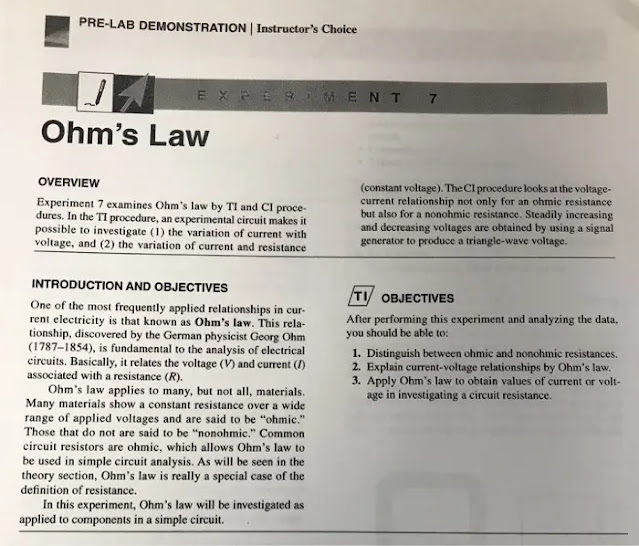### Ohm’s Law Experiment Objectives To verify Ohm’s Law and experimentally, verify the relationship between current, voltage and resistance in a circuit.Ohm’s law – Lab report

### Equipment

#### Resistor Box DC power supply Voltmeter Ammeter

Theory A current is directly proportional to voltage. When a resistance is at stable, is consider to be following the Ohm’s Law. The symbol for ohm or unit is Ω, and this is representing the resistance. Now, in order to comprehend Ohm’s Law is to imagine like a liquid circuit, we have our pump (battery; voltage), then it is flowing and meets it’s resistance. This is the important part of Ohm’s law and the resistance, is that if the resistance is bigger, then less water will flow back. Therefore, given voltage, and depending how big the resistance is, then it will depend how the current will flow through the resistance.

Procedure I. Variation of Current with Voltage when the resistance is constant. 1. First, set up the following circuit shown below, using a power supply, an ammeter (A), a voltmeter (V) and one 1000-Ω Resistor (R) from resistor box. 2. Then, let the instructor check the circuit. 3. Later, close the switch and read the voltage and current on the meters. 4. Next, vary the output voltage of the power supply from 0 to 12 V in the increment of 1.5 V and record the readings of the voltage V across the resistor and corresponding current I through the resistor in Table 1. 5. Repeat this procedure for 1200-Ω Resistor (R) from resistor box. 6. Then, do the graph of the voltage V (vertical axis) versus the electric current I (horizontal axis) for each resistor. 7. Next, calculate the percent error of the actual 1000-Ω Resistor (R) and the experimental 1000-Ω Resistor (R). 8. Finally, repeat step 6 and 7 but for 1200-Ω Resistor (R).

II. Variation of the current with resistance when voltage is constant. 1. First, use the same circuit set up. 2. Next, set the output voltage of power supply to 10 V. 3. Also, by keeping the voltage V across the resistor constant, vary the resistance of the resistor box from 600 Ω to 1200 Ω in increments of 100 Ω. 4. Then, record values of the current I through resistor in Table 2. 5. After that is done, construct a graph of the current I versus the R. Determine the slope of the graph. 6. Next, compare slope of the graph with the constant voltage across the resistors by computing a percent difference.

Analysis From this experiment, we can see that the values of voltages depend on how big are the values of resistors. The bigger the values of resistors, the bigger the values of electromotive force will be resulted. This shows that the voltage range and resistor value is linear to each other, while perpendicular with the value of current.

Conclusion Based on the data collected and the slope calculated in the Voltage vs Current graph of the Rheostat, the percent difference between the slope and the true value of the Rheostat confirms that the experimental value of the Resistance was pretty accurate and that the experiment was successfully accomplished. This is similar to the example of the liner current, the more the resistance the bigger the resistance is the lest water will flow. Ohm’s Law have been confirmed in this laboratory experiment because of the relationship seen between current, voltage, and resistance with the information collected.